# Geometric Points, Lines, and Angles

In geometry, a point has height and width of zero. That is, a point has no length, width, or depth. On the GMAT, in a geometry question, if something looks like a point, it’s usually a point (and it’s generally identified by the question as a point), and you don’t need to second-guess that fact.

A geometric line is as wide as a point, infinitely thin, having an infinite number of points, (in a straight row), extending forever in both the directions. The arrowhead on a line indicates that the line goes on forever: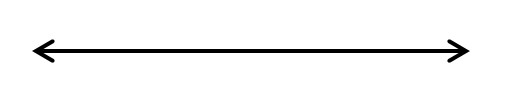On the GMAT, lines are usually coplanar (on the same plane). For example, if you see a figure such as the following, you don’t need to worry about whether it’s a three-dimensional graph or a two-dimensional graph; assume it’s a two-dimensional graph unless you are told otherwise by the question.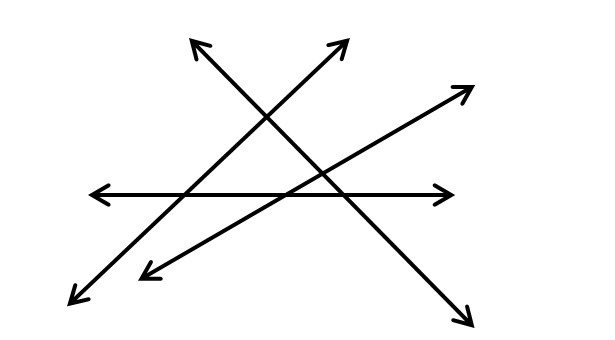Lines are parallel if they do not intersect. And if they do not intersect, then they are parallel. Since lines extend infinitely, if they are not parallel, they will eventually intersect.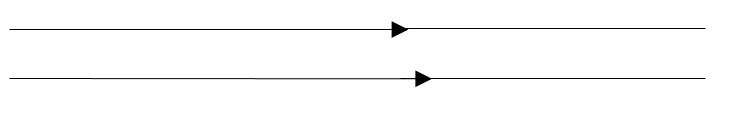GMAT questions usually deal with what are not technically lines, but rather line segments. A line segment, or segment, is a part of a line, which has two endpoints. The endpoints give the line segment a fixed, or finite length. Note that the arrowheads are omitted here, since the line does not extend infinitely: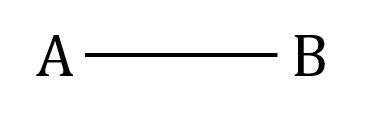Line segments are referred to on the GMAT by their endpoints – for example, as AB and CD. The symbol may have a horizontal bar over it to distinguish the line itself from its length, but the distinction is never practically useful in solving GMAT questions.

There is only a single straight line between two points. Two points uniquely define a line:

##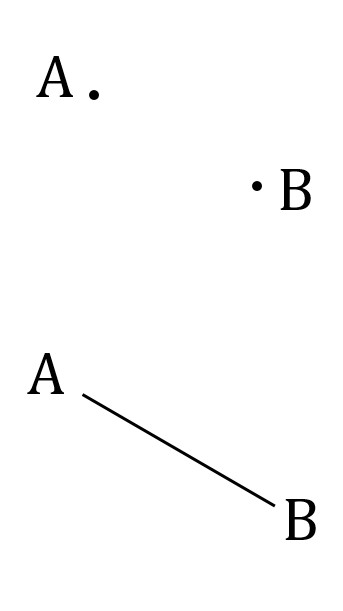Angles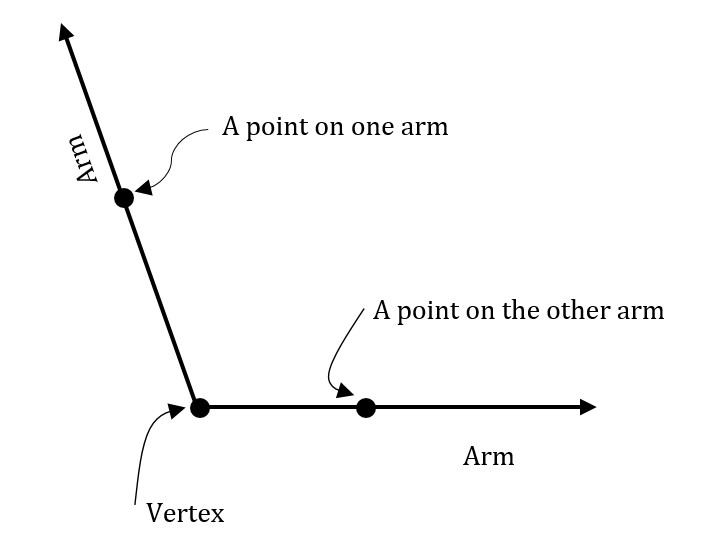An angle is made up of a vertex (a point), two arms (rays), and an arc. The endpoint of the arms is the vertex. The size of the angle depends on how wide the arms are opened, and it is measured in degrees. (Radians, which are favored over degrees in engineering and science to measure angles, are not typically used on GMAT questions.)

An angle that is less than 90 degrees is known as an acute angle. A 90 degree angle is known as a right angle. Those between 90 and 180 degrees are obtuse angles.

Sometimes, two angles may add up to 90 or 180 degrees. They are called complementary angles and supplementary angles, respectively. The terms “complementary” and “supplementary” themselves are not important on the GMAT, but you will frequently deal with angles that add up to 90 or 180 degrees.

## Angles at a Point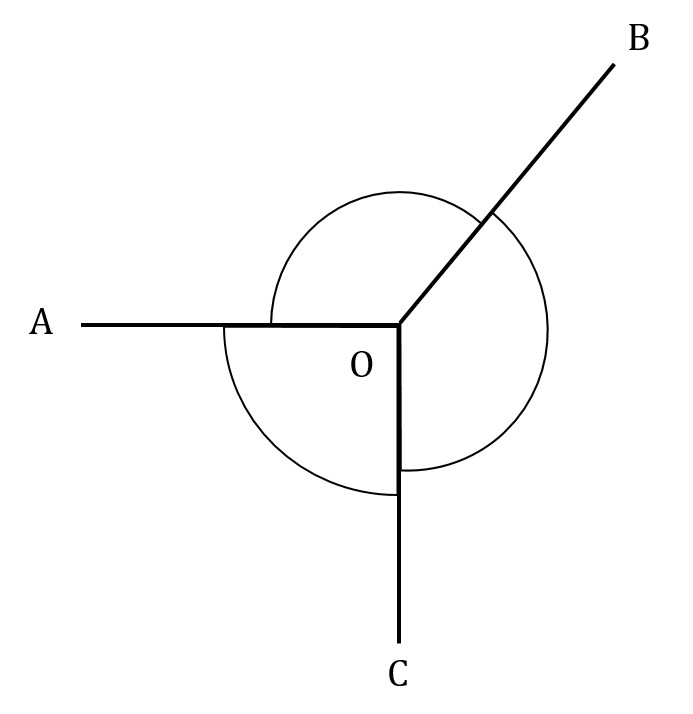Sometimes, two or more angles share a common vertex, and their sizes add up to 360 degrees. Such angles can be called “angles at a point.” For example, in the figure above, the three points, A, B, and C are around the point O. If we knew that angle AOB = 120 degrees and angle BOC = 150 degrees, then we would be able to continue: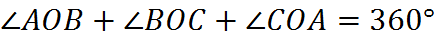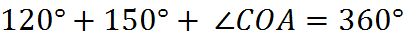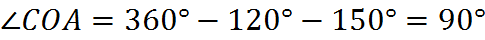## Adjacent Angles on a Straight Line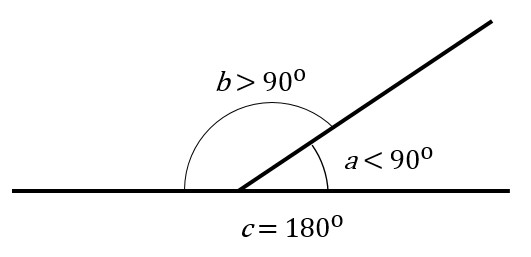When the sizes of adjacent angles add up to 180 degrees, they are adjacent angles on a straight line. They are used when finding out the value of one of the angles.

In the figure above, b and a are supplementary. They are adjacent angles on a straight line; if we know the value of b, we can find out the value of a. Furthermore, the sum of b and a is equal to c. Note that a, b, and c are angles at a point.

## (Vertically) Opposite Angles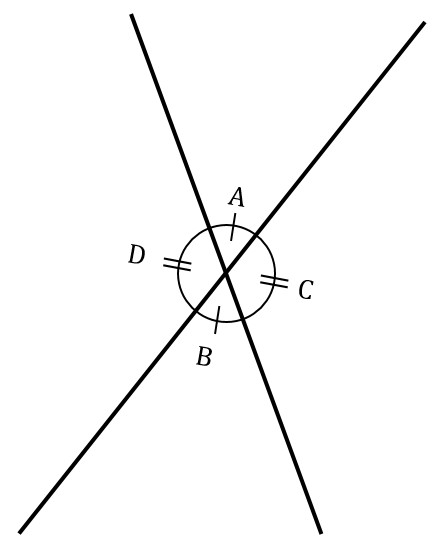Where two lines cross, the opposite angles are equal. You cannot assume that something is a straight line just by observation, but a question will likely refer to it as a line (on most GMAT questions, apparently straight lines are, in fact, straight lines).

As indicated in this figure above, D is equal to C and A is equal to B. This is because they are vertically opposite angles. Here, D and A, A and C, C and B, and D and B are all pairs of adjacent angles on a straight line. Also, the four angles are angles at a point.

## Transverse Angles

A transversal is a line segment that cuts through two line segments. When the two line segments are parallel, the eight angles produced will have some special properties. The angles will be equal as indicated by this diagram: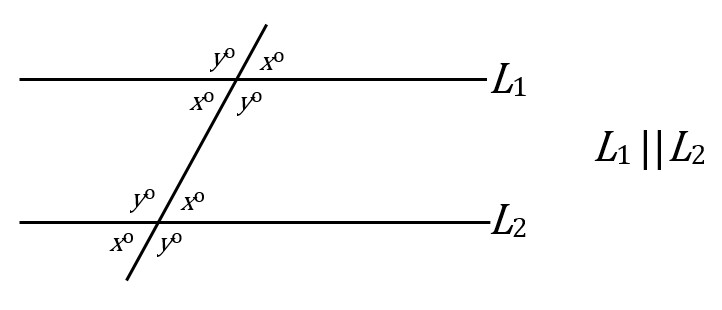Let us look at an example. In the figure below, given that DE and BH are parallel, how can we find x, y and z?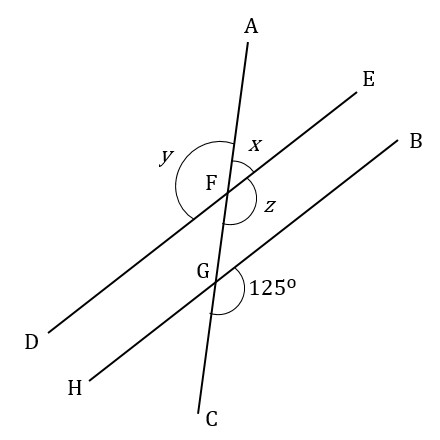Since the lines DE and BH are parallel, we can equate these two “corresponding” angles: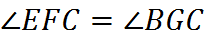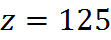We can equate ERC to the vertically opposite angle AFD. And since we just found that angle EFC is equal to angle BGC, that means its vertical angle also equals angle BGC (as it always will):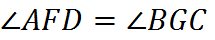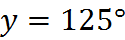We have found first z, then y. We can find the value of x from either one, because it is supplementary to a line with either one of the (equal) angles. For example,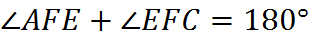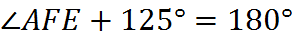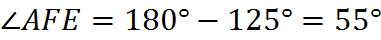Welcome! You are encouraged to register with the site and login (for free). When you register, you support the site and your question history is saved.